Select Page## Web spreadsheet, a use case in EMS

Web spreadsheet, used in the context of IoT

### What is this all about?

In spring 2020 the beginning of the cooperation between the German green-tech startup Energenious GmbH and the Italian IoT specialist Tecnojest srl was announced. At the core of the cooperation there is the design of an efficient architecture to develop, integrate, and distribute UI modules for data-centric web applications. The new architecture should be easy to understand for web developers and allow for quick integration of new UI modules (Widgets) in a “puzzle-like” fashion.

This new concept was given the name of “Widget-driven design”. You can read more about it in my previous article.

The project is still very much active and the 2 companies have come a long way in research and implementation of an open source JavaScript library to develop and use Widgets in innovative applications for data analysis.

In this article, I will give an overview of one of a series of interesting Widgets that have been developed using the open source library from above: the so-called Web spreadsheet (WSS). This article explores the basic functionality of WSS and gives a simple example of how it can be used in the context of Energy management systems (EMS).

Note: the next section is somewhat theoretical, so if you want to see some real-life usage of WSS you can jump to the end of the article.

And now, let the work begin!

### WSS — The basics

So what is WSS? Well, the name tells it:

WSS is a web implementation of an interactive spreadsheet that allows for simple data analysis and visualization of both real time and historical data

At its core, WSS consists of an interactive matrix and a toolbar with styling options. The matrix is where the user spends most of the time, and its basic component is the Cell.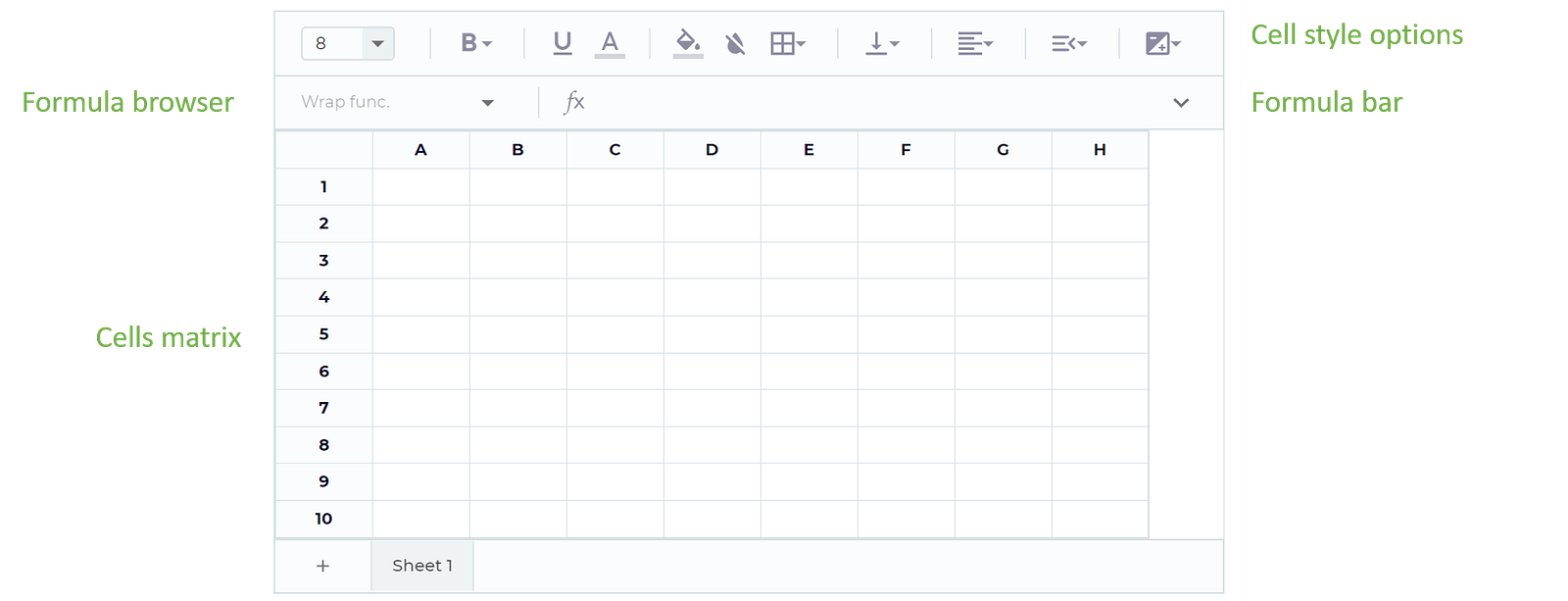Basic structure of WSS

#### The anatomy of a Cell

The basics of WSS are straight forward to grasp for users already experienced with tools such as Microsoft Excel or Open Office, as it builds on a similar UX. WSS is, however, completely web-based, thus it runs in a regular web browser and requires no extra software installation.

The basic component of WSS is the Cell, consisting of 2 main properties:

• a Formula: e.g. `=SUM(A1:A3)`
• a Style: e.g. `color: red`

Cells are styled using regular CSS rules, which are quite rich in nature. Cell styles can also be dependent on the Cell’s value, but this is a topic for another post.

Formulas are, instead, defined in 2 kinds: default and custom formulas. WSS starts with a set of default formulas, which includes operators for performing simple math, and string or date manipulation, such as `SUM`, `ACOS`, `CONCATENATE`, `DATE`, etc. In addition to this, WSS offers a simple JavaScript based API, for developers to define their own custom formulas. Here is an example for how a developer can define a very simple formula, named `NOW`, to return the current date and time using JavaScript Objects:

``````{
name: "NOW",
description: "Returns the current day and time",
fn: () => (new Date()).toISOString()
}
``````

To invoke the formula in WSS, it is enough to call it in the spreadsheet’s formula bar using the syntax: `=NOW()`. Here is the outcome: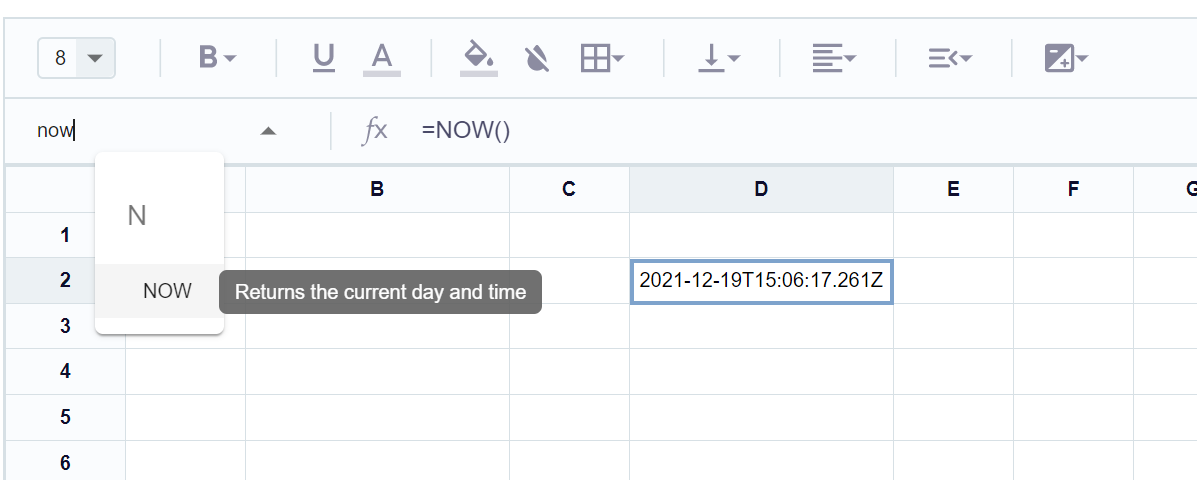How to call a custom formula named “NOW”

In the example above you can see the formula invocation `=NOW()` in the formula bar (center top), the output of the formula in cell D2, and the description of the formula on the specific element of the dropdown menu (top left). The dropdown menu, also called the Formulas browser, displays the list of all available formulas, filtered by name.

Formulas can also accept parameters, which could be constant arguments, references to other cells, or even nested formulas. Custom and default formulas can be also used together, for instance to concatenate strings: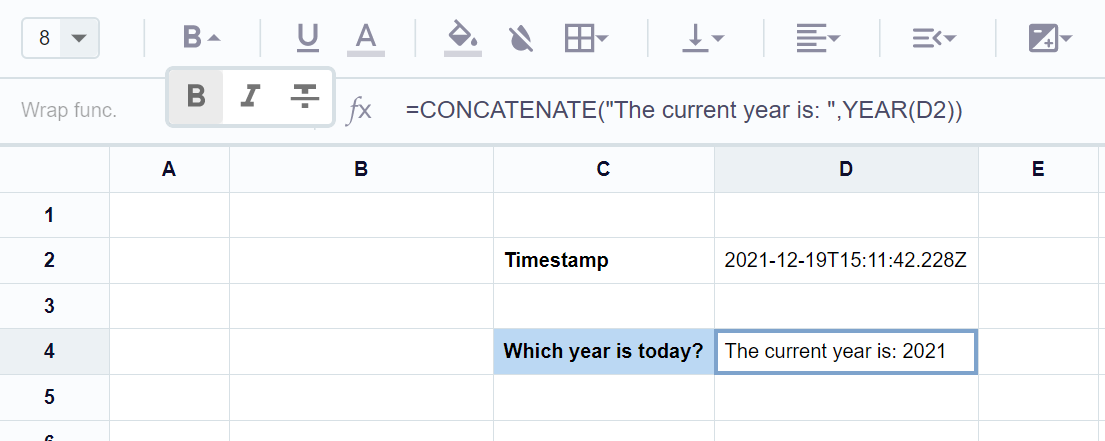Example of nested formulas and cell references

The formula in D4 receives 2 parameters: a constant string `The current year is:`, and a nested formula `YEAR(...)`, which in turns extracts the year from the timestamp in D2. In the example above, some font styling and coloring to cells C2 and C4 were added, to show basic cell styling.

Accessing parameters within custom formulas, is as simple as getting them from the definition of the `fn` attribute. Here is how to define a formula using TypeScript, to display the timestamp of the past “N” hours, where “N” is a user defined parameter:

``````{
name: "LAST_HOUR",
fn: (params: any[]) => {
let hoursAgo = params ?? 1;
let time = new Date(Date.now() - 1000 * 60 * 60 * hoursAgo);
return time.toISOString();
}
}
``````

#### More complex use cases

In the previous section, you could get a glimpse of basic UX in WSS, from both User and Developer’s point of view. Now we can proceed with some more interesting use cases for formulas.

Each Cell belongs to one of four categories, according to the data structure returned by the `fn` function:

• Constant: the cell holds no real formula, i.e. the cell is a simple text, not starting with the special character `=`. E.g. `"Hello world"`
• Variable (synchronous) : The formula returns a value, immediately after its execution. This is the case for all default formulas, such as the ones seen in the examples above: e.g. `=NOW()`
• Variable (asynchronous): The formula returns a JavasScript Promise. While the Promise is still pending, the Cell displays the special value `#LOADING`. When the Promise resolves, the Cell displays its return value, or `#ERROR` in case of exceptions
• Variable (real-time): The formula returns no direct value, but it binds the Cell to an event listener (for example a TCP socket). When the listener triggers, the callback defined in the formula is executed and its result displayed in the Cell to the user

While real-time Cells are still under development, all other kinds are already available in the most stable WSS release (version 1.7 as of today).

The possibility of using customized, asynchronous formulas makes WSS very powerful in data-driven applications. In the next section I will give some interesting examples.

### WSS, a use case in energy management systems (EMS)

Remember:

Web spreadsheet (WSS) is a Widget_, this means that it can be used both as a simple standalone web application, or it can be_ integrated in third-party environments using the same API

In the following use case, we have embedded WSS within an IoT platform owned by the Italian company Tecnojest srl. The platform manages a big amount of data coming from field devices gathering, among others, historical information about energy systems. Such information includes data such as electric voltage, power, current, etc. and is stored in an OLAP backend. These data are then made available via a web API to data visualization framework, to make queries and produce diagrams.

To integrate WSS in Tecnojest’s platform, developers have created a set of custom formulas, which are specific for the IoT domain, and allow the user to perform quick data analysis, without the need of knowing any query language.

This prototype was used to showcase how WSS can deliver very useful information to its stakeholders about the state of an energy system at a specific point in time. This way, the user can get answers to question such as:

• what was the total energy consumption in the office last week?
• how high should I expect the next energy bill to be?
• what was the total energy consumption from day X to day Y last year?

Retaining such kind of information can be done in just 5 minutes using WSS.

Here I’ll show you how.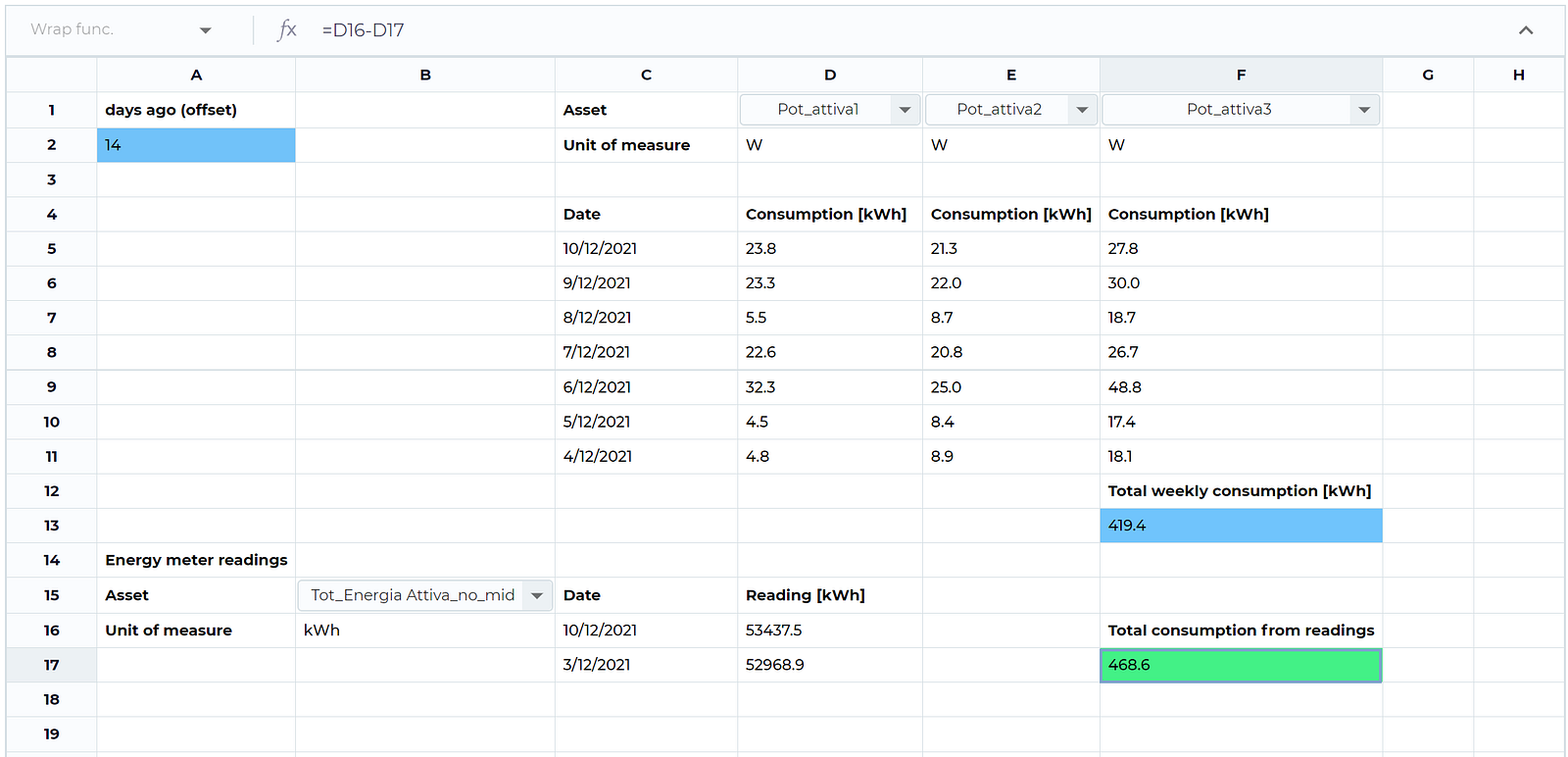Preview of what we will achieve in this tutorial

#### The setup

Before exploring how WSS is capable to retrieve and display energy related information, here a quick primer on EMS:

• electrical energy: it is typically measured in kilowatt hours (kWh). Energy meters keep count of the total energy consumed over the time, thus one could derive the energy used on a specific day by just taking the difference between the count of 2 consecutive days
• point of delivery (POD): this is usually the place where the energy system connects to the main power grid. In typical buildings this is also the place the whole electrical energy is drawn from, and which is paid for periodically in the energy bill
• electric power: it is measured in Watts (W). Power can be used to derive the total energy consumption by taking its time integral over a given period
• energy asset: by asset we mean any kind of equipment (metering infrastructure, actuators, and others) whose data can be stored in Tecnojest’s IoT platform and can be accessed using a unique identifier (ID)

Given this core “domain knowledge” we are now capable of performing basic data analysis on a simple energy system consisting of 3 power meters (output in Watt) and an energy meter, mounted at the POD of a small office.

We will proceed in these steps:

1. define a time period for the analysis, say one week
2. fetch historical data from the relevant asset (power and energy meters)
3. aggregate the data to compute energy consumption

To simplify the user workflow, some custom (asynchronous) formulas for those “domain-specific” operations have been defined. Those are:

• `DEVICES()`: returns a list of all available asset in the EMS, as a dropdown selector. Selecting one element will return the ID of the respective asset
• `DEVICE.UNIT(id: int)`: given the ID of an asset, returns its registered unit of measure, if it exists
• `DEVICE.LAST_DAY(id: int, offset?: int = 1)`: given the ID of an asset, returns the average measured value in the past 24 hours. The variable `offset` allows users to average over more days in the past
• `DEVICE.LAST_DAY.MAX(id: int, offset?: int = 1)`: this formula works like the previous one, but it returns the highest value measured in the past 24 hours, instead of the average value

With these custom formulas available to the user, it is quite simple to estimate the total weekly consumption in the energy system. Let’s see how.

#### Step 1 — define a time step for the analysis

this step is as simple as defining one cell in WSS whose value will be used as the `offset` value of the `DEVICE.LAST_DAY` formula: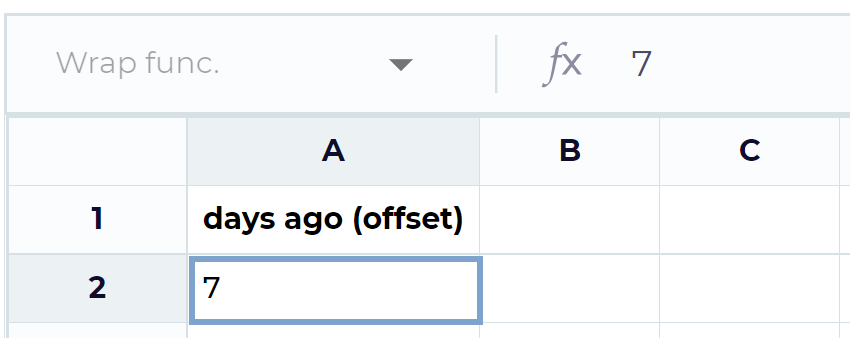Defining a constant Cell in WSS

The `offset`parameter will then be incremented according to the which day we want to fetch the data for.

#### Step 2 — fetch historical data from the relevant asset

This step involves 2 sub-steps: finding the right asset ID, and then fetching the actual data.

To achieve this, we first call the `DEVICES` formula (cells D1, E1, F1) and then use its output, the selected asset ID, as the first argument of the `DEVICE.LAST_DAY` formula (D14, E4, F4). To check that we have picked to correct asset, we fetch also the unit of measure of the asset by using `DEVICE.UNIT`(D2, E2, F2). This is Watt (W) in case of power meters.

Here the results: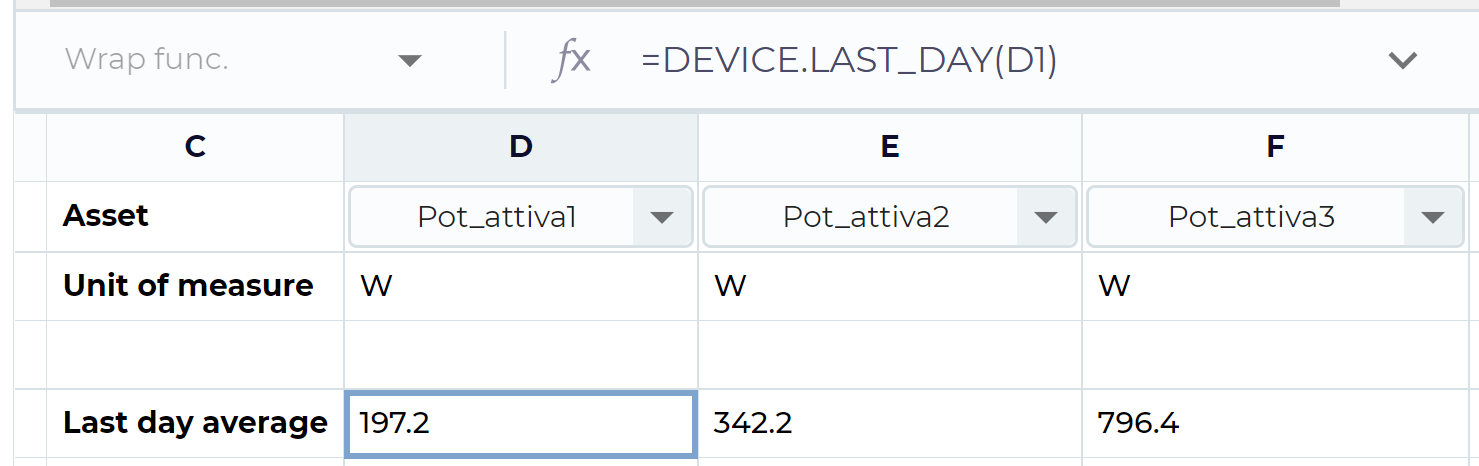Fetching last 24 hours of data for specific energy asset

In order to convert power values into energy we simply have to multiply the average hourly power times 24 (hours in a day). This means a small change in the formulas in cell D4 to F4:

``````=DEVICE.LAST_DAY(E1)*24
``````

This leads to the daily consumption in Watthours (Wh).

Once we have extracted the desired information for one day, we just need to replicate and adapt the formula for to the remaining 6 days, so we can derive the total energy consumption for the whole week. In order to keep track of the single days, we can also add a column with a human-readable date indication using operators for date/string manipulation. This is the outcome of this step: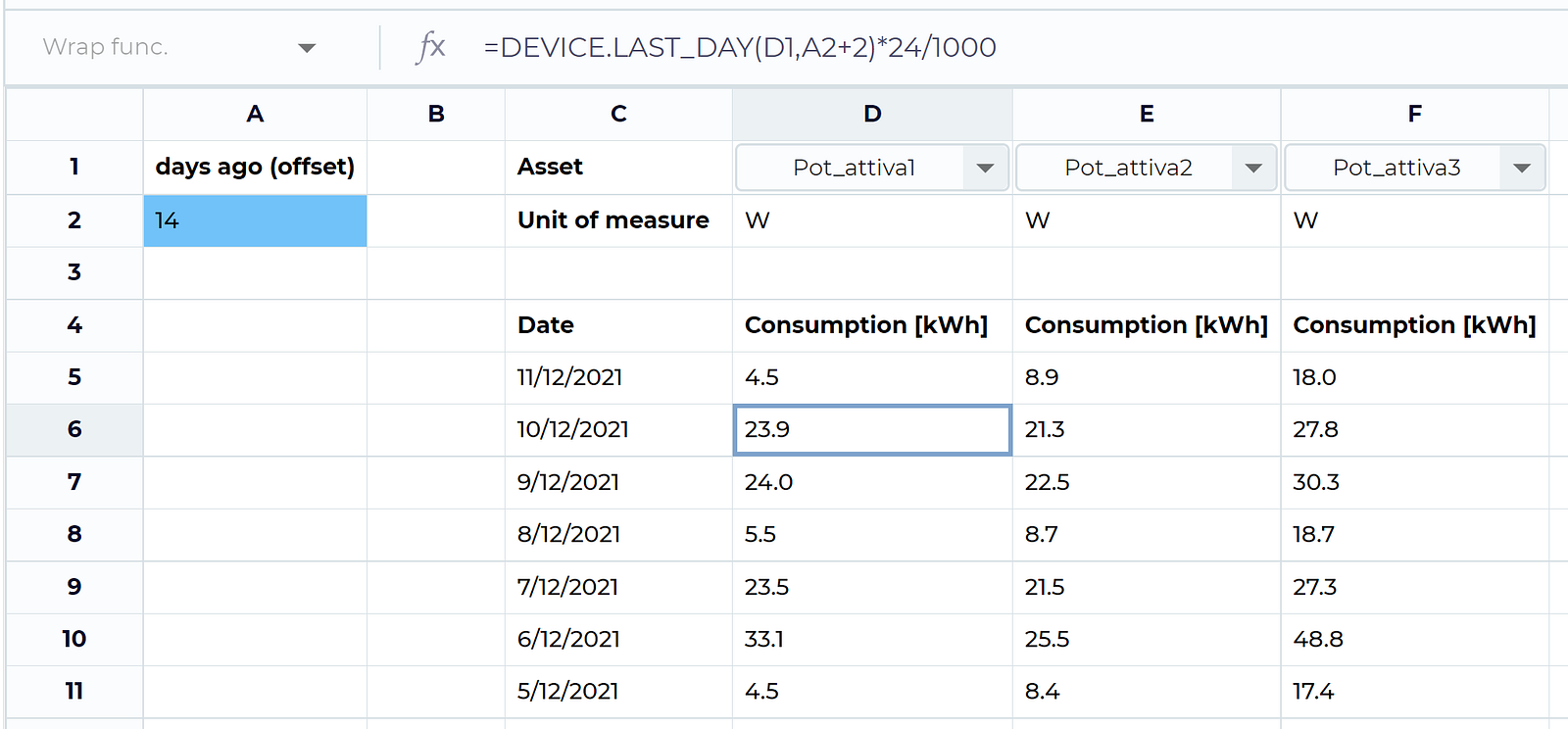Defining a human-readable data frame of daily energy consumption from 3 weeks ago

#### Step 3 — aggregate the data to compute energy consumption

This is perhaps the easiest part, the only operation needed is to call the default formula `SUM` and reference the cell ranges we are interested to get the sum from, like this (cell F13):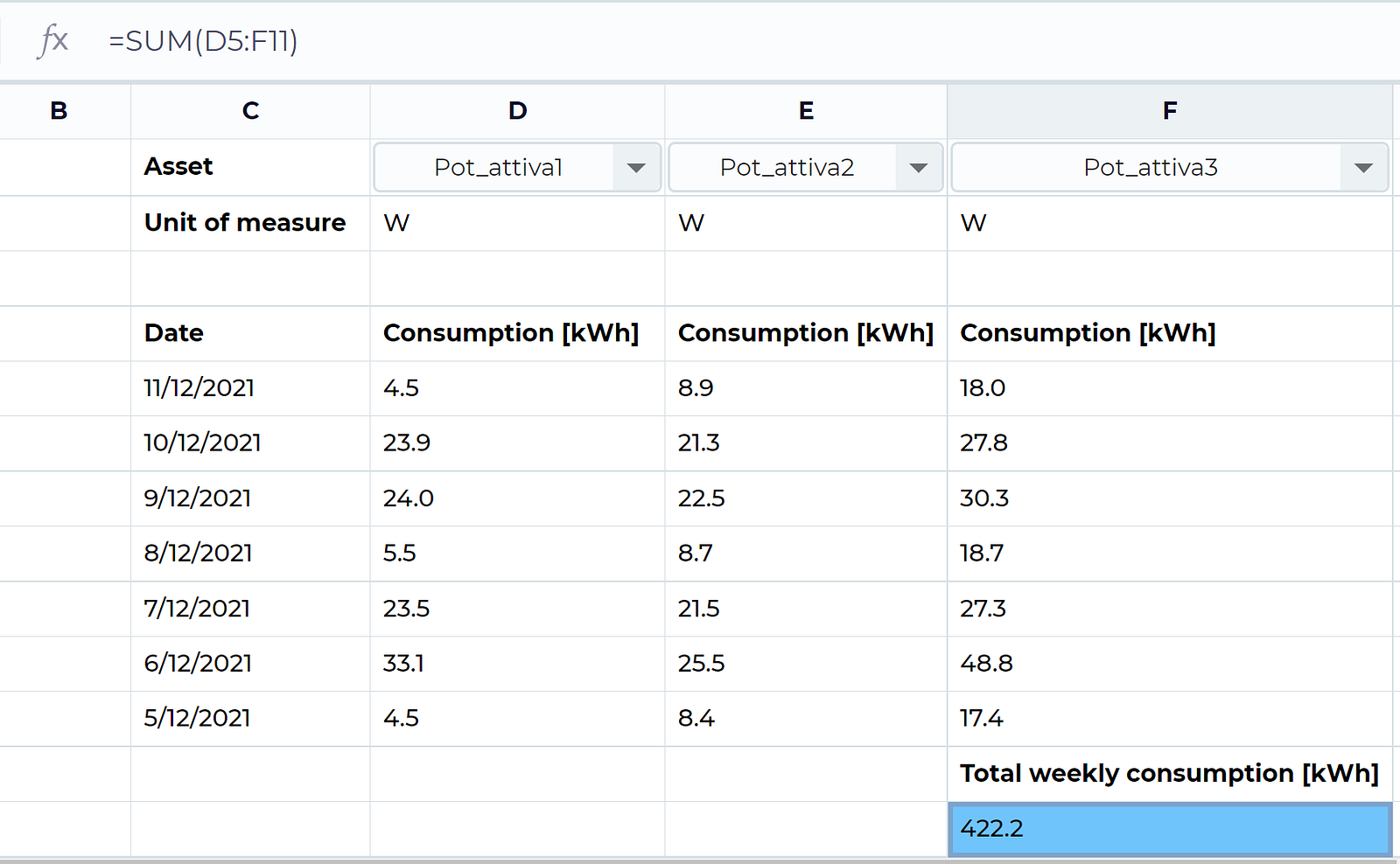Summing over a range of rows/columns

### Validating the results

As you could notice in the previous section, setting up WSS for aggregating the historical information about field devices is quite straight forward. Here we want to go on step deeper and validate that estimated total energy consumption is correct. To do this we will rely on the historical data from the energy meter, mounted at the POD.

As stated above, deriving energy consumption from the energy meter is as simple as getting the difference between two subsequent readings; but there is one catch: to be precise with the calculation we cannot use the average measured value, like we did for the electric power. Instead, we have to take exactly the first value from the meter at the beginning of the week and subtracting it from exactly the last value measured at the end of the week. Again, thanks to our domain knowledge, we know that energy meters “count energy”, thus their value must be strictly increasing.

To obtain the needed data from the meter, we can therefore use the `DEVICE.LAST_DAY.MAX` function on a specific day, and get the last value measured on that day, which is indeed the “highest” one. And so, to get the whole energy consumption from last week, from an energy meter with, e.g. ID 123, we can use this simple formula:

``````DEVICE.LAST_DAY.MAX(123, 1) - DEVICE.LAST_DAY.MAX(123, 8)
``````

This is best achieved in WSS by splitting this formula over 3 cells, as in cell F17 (look at the formula bar):Validating indirect consumption estimate (F13) against actual energy meter data (F17)

If we have a closer look at the energy values derived from the sum over all power meters (indirect estimate in F13) and the ones extracted from the energy meter (direct estimate in F17) we find an error of about 10 % when using the indirect method. This can be due to averaging errors such as statistical skew of the power measurements. I could write an article in future, about how to reduce this error, but since this is very domain-specific, I would first like to hear about your interest in the comment section, so reach me out at daniele@energenious.eu in case.

### Lessons learned and way forward

Throughout the last year and a half our team has gathered deep knowledge on Widget-driven development and has come a long way implementing functional prototypes in real life production environments. We recognize the potential of this very modular design pattern, but have also become much more aware of the challenges during integration of widgets in foreign environments.

Enriched by this experience, we have decided to devote our efforts in reducing the burden that developers may encounter during ideation, integration, and customization of new, or existing widgets.

WSS is a clear example of how domain knowledge can be easily integrated into a very user-friendly UI module thanks to a standardized DSL, based on custom formulas.

### Conclusion

In this article, the simple, yet powerful concept of custom formulas in WSS was introduced. This concept was then applied to perform basic data analysis for energy management systems.

The material covered in this article barely scratches the surface of potential WSS application. We are currently working on various use cases in the fields of water network management, telecommunication, and industrial IoT.

I am planning to publish more articles showcasing more advanced features of WSS. Those include:

• Data and state (de-)serialization in WSS
• How to analyze GIS data using WSS
• How to define custom styles
• How to define WSS to track Key performance indicators
• Use of real-time cells and dynamic cell formatting
• How to export WSS cells as Lambda functions

As you can see, the list is already pretty comprehensive, but we are continuously looking forward to hear your feedback and your preference in terms of use cases and features that should be covered next.

Let me know at daniele@energenious.eu or in the comment section.

The material covered in this article is a result of the ongoing cooperation between the Italian Tecnojest srl and the German Energenious GmbH who jointly provide cloud based solutions for a more energy efficient future.